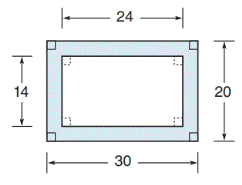Chapter 8.1, Problem 19EElementary Geometry For College St...

7th Edition
Alexander + 2 others
ISBN: 9781337614085

Solutions

Chapter
SectionElementary Geometry For College St...

7th Edition
Alexander + 2 others
ISBN: 9781337614085
Textbook Problem

In Exercises 19 to 22, find the area of the shaded region.To determine

To find:

The area of the shaded region.

Explanation

Finding the area of the figure is followed by identifying the figure and find area with the suitable geometry formulae.

Calculation:

Given,

Which are rectangle.

Length of the outer rectangle l1=30

Width of the outer rectangle w1=20

Length of the inner rectangle l2=24

Width of the inner rectangle w2=14

Area of the shaded region (A)=Area of outer rectangleArea of inner rectangle

A=l</

Still sussing out bartleby?

Check out a sample textbook solution.

See a sample solution

The Solution to Your Study Problems

Bartleby provides explanations to thousands of textbook problems written by our experts, many with advanced degrees!

Get Started

In Exercises 2336, find the domain of the function. 32. f(x)=1x2+x2

Applied Calculus for the Managerial, Life, and Social Sciences: A Brief Approach

Convert the expressions in Exercises 6584 to power form. x2x

Finite Mathematics and Applied Calculus (MindTap Course List)

In Problems 47-50, rationalize the denominator of each fraction and simplify. 48.

Mathematical Applications for the Management, Life, and Social Sciences

If 13f(x)dx=10 and 13g(x)dx=6, then 13(2f(x)3g(x))dx= a) 2 b) 4 c) 18 d) 38

Study Guide for Stewart's Single Variable Calculus: Early Transcendentals, 8th